Published

MEGR-3171 Grease Fire Extinguishing Sprinkler System

Forgot the stove on again? Don't worry! This system stops grease fires in their tracks. By: Willie Grant, Rubicel Villeda, Prince Muhumur

IntermediateFull instructions provided3 hours624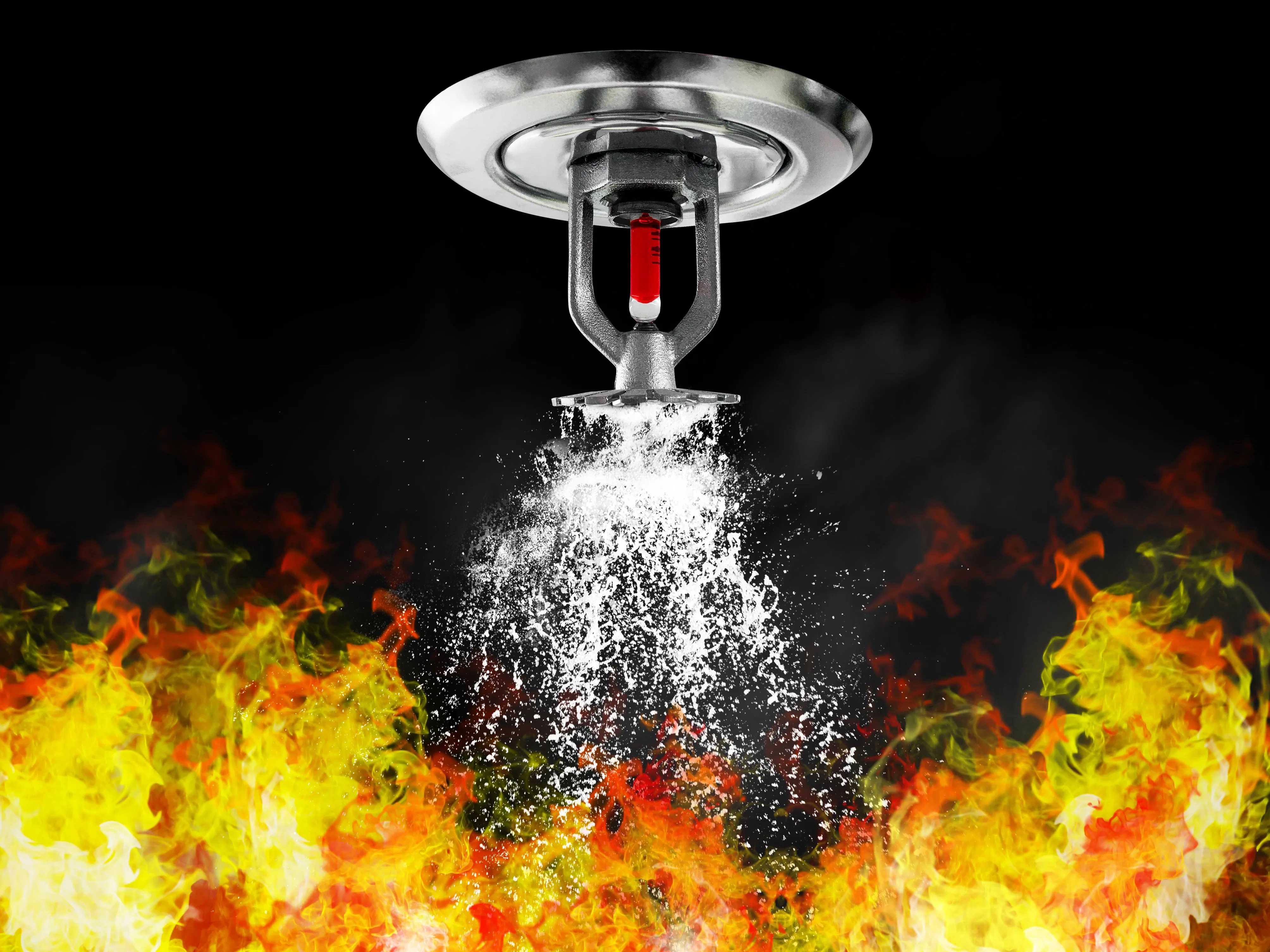Things used in this project

Hardware componentsParticle Photon
×3
 DC motor (generic)
×1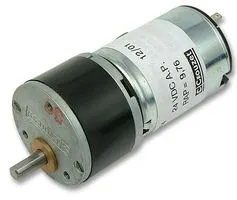Geared DC Motor, 12 V
×1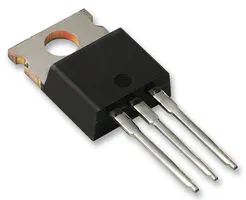Darlington High Power Transistor
×1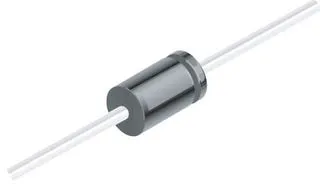1N4007 – High Voltage, High Current Rated Diode
×1LED (generic)
×2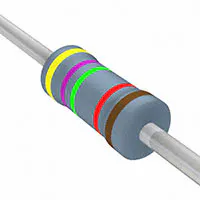Resistor 47.5k ohm
×1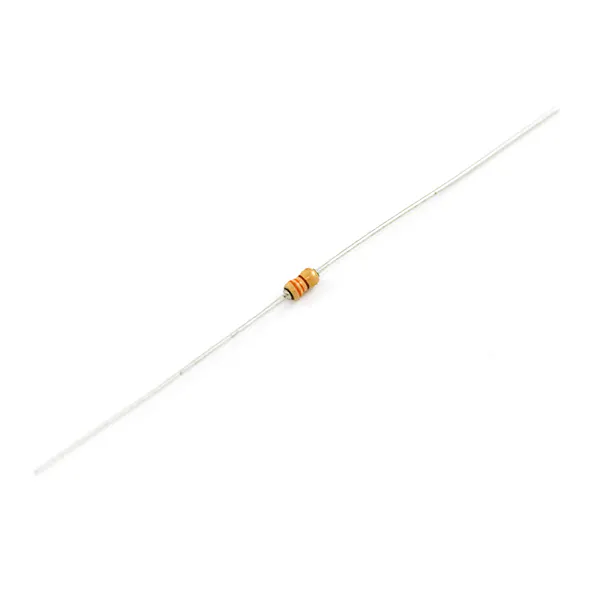Resistor 330 ohm
×2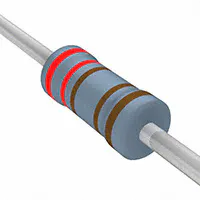Resistor 2.21k ohm
×1Jumper wires (generic)
×1×1

Software apps and online servicesParticle Build Web IDEIFTTT Maker serviceSchematics

Sprinkler Schematic

Thermistor is connected to analog pin A0 and reads its value. Photon tells the motor to turn on using digital pin D0 transistor and diode. Motor is powered by 12 volts and plugs into wall outlet.

Manual Remote Schematic

Button switch is connected to digital pin D2. LED is connected to digital pin D0. When button is pressed down, digital pin D2 reads the state/status of the button.

Notifier Schematic

A LED is connected to digital pin D5 and turns on when it receives notice that an event from either of the other photons has been published.

Code

Manual Remote Code

C/C++
int led = D0;
int button = D2;
unsigned long interval = 0;
int howlong = 10000;
int temperature_ifttt;
String ledState = "off";

void setup() {
Serial.begin(9600);
pinMode(button, INPUT_PULLUP);
pinMode(led, OUTPUT);
digitalWrite(led, LOW);
}

void loop() {
if( (millis() - interval > howlong) or (interval==0) ) {
interval = millis();
}
if(buttonState == LOW) {
if(ledState == "off") {
ledState = "on";
digitalWrite(led, HIGH);
Particle.publish("photonchat", "Hey, Hows the photon life");
}
else {
ledState = "off";
digitalWrite(led, LOW);
}
}
delay(200);
}

Sprinkler Code

C/C++
// This #include statement was automatically added by the Particle IDE.
#include <ThingSpeak.h>
#include <math.h>
#include "application.h"

#define THERMISTORNOMINAL 3000
#define TEMPERATURENOMINAL 21.11
#define NUMSAMPLES 5
#define BCOEFFICIENT 3950
#define SERIESRESISTOR 10000
#define publish_delay 16000

bool COLDROOM= false;
bool HOTROOM = false;
bool useFahrenheit = true;

unsigned int lastPublish = 0;
unsigned long interval = 0;
int samples[NUMSAMPLES];
int THERMISTOR = A0;
int motor = D0;
int _version = 1;
char temperature_str;
char temperature_ifttt;

void setup() {

pinMode(motor, OUTPUT);
digitalWrite(motor, LOW);
Particle.subscribe("photonchat", anything, "300038000647373034353237");

interval = 0;
Particle.function("status", status);
pinMode(THERMISTOR, INPUT);

Particle.variable("tmp", temperature_str, STRING);
}

void anything(const char *event, const char *data){
digitalWrite(motor, HIGH);

}
void loop(){
if( (millis() - interval >= READ_INTERVAL) or (interval==0) ) {
temp();
interval = millis();
}
}

int status(String args)
{

Particle.publish("temp", temperature_ifttt, 60, PUBLIC);
return 0;
}

int temp()
{
uint8_t i;
float average;
for (i=0; i< NUMSAMPLES; i++) {
delay(10);
}
average = 0;
for (i=0; i< NUMSAMPLES; i++) {
average += samples[i];
}
average /= NUMSAMPLES;
average = (4095 / average)  - 1;
average = SERIESRESISTOR / average;

float steinhart;
steinhart = average / THERMISTORNOMINAL;
steinhart = log(steinhart);
steinhart /= BCOEFFICIENT;
steinhart += 1.0 / (TEMPERATURENOMINAL + 273.15);
steinhart = 1.0 / steinhart;
steinhart -= 273.15;

if (useFahrenheit) {
steinhart = (steinhart * 9.0)/ 5.0 + 32.0;
}
char ascii;
int steinhart1 = (steinhart - (int)steinhart) * 100;
steinhart1 = abs(steinhart1);
sprintf(ascii,"%0d.%d", (int)steinhart, steinhart1);
Particle.publish("Room_Temperature", ascii, 60, PRIVATE);
char tempInChar;
sprintf(tempInChar,"%0d.%d", (int)steinhart, steinhart1);
sprintf(temperature_str, "{\"t\":%s}", tempInChar);
sprintf(temperature_ifttt, "%s", tempInChar);

if( steinhart > 90){
Particle.publish("HOTROOM", "True");
digitalWrite(motor, HIGH);
Particle.publish("photonlife", "Hey, Hows the photon life");
} else {
Particle.publish("COLDROOM", "True");
digitalWrite(motor,LOW);

}
return 0;
}

Notifier Code

C/C++
int LED = D5;

void setup(){
pinMode(LED, OUTPUT);
Particle.subscribe("photonchat", anything, "300038000647373034353237");
Particle.subscribe("photonlife", anything, "45002e000647373034353237");
}
void anything(const char *event, const char *data)
{
digitalWrite(LED, HIGH);
}

Credits

Rubicel Trejo

0 projects • 2 followers

Prince Muhumure

0 projects • 1 follower